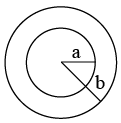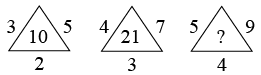# SSC JE Electrical Engineering 25th May 2014 Shift-1

Instructions

For the following questions answer them individually

Question 31

# If radius b is double that of radius a, the area of the smaller circle to that of the larger circle is in proportion :Instructions

Insert the arithmetic signs in the following numerical figure:

Question 32

Question 33

# 9, 3, 4, 6 = 29

Instructions

For the following questions answer them individually

Question 34

# If 7x - 5y = 20 and 12x + 5y = 75, what is the value of xy?

Instructions

In the following questions, select the missing number from the given responses.

Question 35

Question 36

Question 37

#Instructions

For the following questions answer them individually

Question 38

Question 39

Question 40

OR